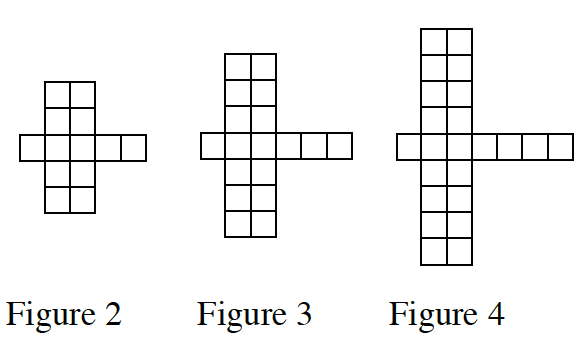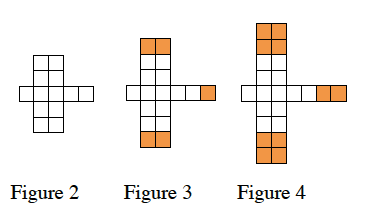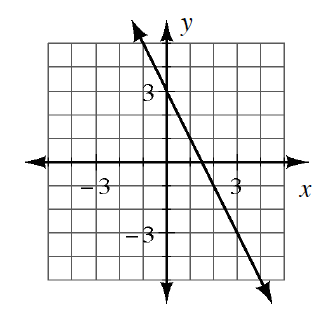### Home > CC3MN > Chapter 5 Unit 6 > Lesson INT1: 5.1.3 > Problem5-39

5-39.

Each part (a) through (d) below represents a different tile pattern. For each, find the pattern of growth and the number of tiles in Figure 0.

1.How many boxes does each figure increase by?Pattern of growth: $5$
Make a sketch of figure 1, then figure 0.
How many tiles does Figure 0 have?

1.Find the slope of the line.

What is $y$ when $x=0$?

Pattern of growth: $−2$
Figure 0: $3$

1. $y=3x−14$

What is the growth factor for the line?

See part (b).

1.  $x$ $-3$ $-2$ $-1$ $0$ $1$ $2$ $3$ $y$ $18$ $13$ $8$ $3$ $-2$ $-7$ $-12$

 $x$ $-3$ $-2$ $-1$ $0$ $1$ $2$ $3$ $y$ $18$ $13$ $8$ $3$ $-2$ $-7$ $-12$ $\color{orange}{-5}$            $\color{orange}{-5}$          $\color{orange}{-5}$         $\color{orange}{-5}$           $\color{orange}{-5}$           $\color{orange}{-5}$